moveit2 The MoveIt Motion Planning Framework for ROS 2.
ompl_interface::BoxConstraint Class Reference

Box shaped position constraints. More...

`#include <ompl_constraints.h>`

Inheritance diagram for ompl_interface::BoxConstraint: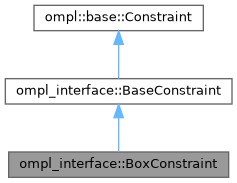[legend]
Collaboration diagram for ompl_interface::BoxConstraint: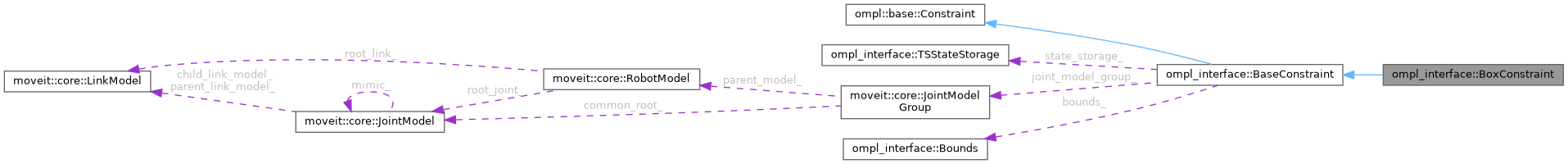[legend]

## Public Member Functions

BoxConstraint (const moveit::core::RobotModelConstPtr &robot_model, const std::string &group, const unsigned int num_dofs)

void parseConstraintMsg (const moveit_msgs::msg::Constraints &constraints) override
Parse bounds on position parameters from MoveIt's constraint message. More...

Eigen::VectorXd calcError (const Eigen::Ref< const Eigen::VectorXd > &x) const override
For inequality constraints: calculate the value of the parameter that is being constrained by the bounds. More...

Eigen::MatrixXd calcErrorJacobian (const Eigen::Ref< const Eigen::VectorXd > &x) const override
For inequality constraints: calculate the Jacobian for the current parameters that are being constrained.

• This error jacobian, as the name suggests, is only the jacobian of the position / orientation / ... error. It does not take into account the derivative of the penalty functions defined in the Bounds class. This correction is added in the implementation of of BaseConstraint::jacobian.
More...Public Member Functions inherited from ompl_interface::BaseConstraint
BaseConstraint (const moveit::core::RobotModelConstPtr &robot_model, const std::string &group, const unsigned int num_dofs, const unsigned int num_cons=3)
Construct a BaseConstraint using 3 `num_cons` by default because all constraints currently implemented have 3 constraint equations. More...

void init (const moveit_msgs::msg::Constraints &constraints)
Initialize constraint based on message content. More...

void function (const Eigen::Ref< const Eigen::VectorXd > &joint_values, Eigen::Ref< Eigen::VectorXd > out) const override

void jacobian (const Eigen::Ref< const Eigen::VectorXd > &joint_values, Eigen::Ref< Eigen::MatrixXd > out) const override
Jacobian of the constraint function. More...

Eigen::Isometry3d forwardKinematics (const Eigen::Ref< const Eigen::VectorXd > &joint_values) const
Wrapper for forward kinematics calculated by MoveIt's Robot State. More...

Eigen::MatrixXd robotGeometricJacobian (const Eigen::Ref< const Eigen::VectorXd > &joint_values) const
Calculate the robot's geometric Jacobian using MoveIt's Robot State. More...

const Eigen::Vector3d getTargetPosition ()

const Eigen::Quaterniond getTargetOrientation ()Protected Attributes inherited from ompl_interface::BaseConstraint
TSStateStorage state_storage_
Thread-safe storage of the robot state. More...

const moveit::core::JointModelGroupjoint_model_group_

Robot link the constraints are applied to. More...

Bounds bounds_
Upper and lower bounds on constrained variables. More...

Eigen::Vector3d target_position_
target for equality constraints, nominal value for inequality constraints. More...

Eigen::Quaterniond target_orientation_
target for equality constraints, nominal value for inequality constraints. More...

## Detailed Description

Box shaped position constraints.

Reads bounds on x, y and z position from a position constraint at constraint_region.primitives.dimensions. Where the primitive has to be of type `shape_msgs/SolidPrimitive.BOX`.

These bounds are applied around the nominal position and orientation of the box.

Definition at line 257 of file ompl_constraints.h.

## ◆ BoxConstraint()

 ompl_interface::BoxConstraint::BoxConstraint ( const moveit::core::RobotModelConstPtr & robot_model, const std::string & group, const unsigned int num_dofs )

Definition at line 191 of file ompl_constraints.cpp.

## ◆ calcError()

 Eigen::VectorXd ompl_interface::BoxConstraint::calcError ( const Eigen::Ref< const Eigen::VectorXd > & x ) const
overridevirtual

For inequality constraints: calculate the value of the parameter that is being constrained by the bounds.

In this Position constraints case, it calculates the x, y and z position of the end-effector. This error is then converted in generic equality constraints in the implementation of `ompl_interface::BaseConstraint::function`.

This method can be bypassed if you want to override `ompl_interface::BaseConstraint::function directly and ignore the bounds calculation.

Reimplemented from ompl_interface::BaseConstraint.

Definition at line 214 of file ompl_constraints.cpp.

Here is the call graph for this function: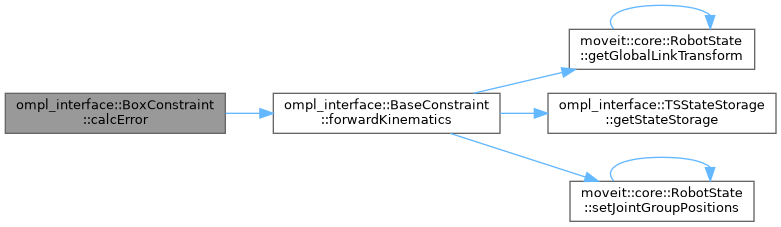## ◆ calcErrorJacobian()

 Eigen::MatrixXd ompl_interface::BoxConstraint::calcErrorJacobian ( const Eigen::Ref< const Eigen::VectorXd > & x ) const
overridevirtual

For inequality constraints: calculate the Jacobian for the current parameters that are being constrained.

• This error jacobian, as the name suggests, is only the jacobian of the position / orientation / ... error. It does not take into account the derivative of the penalty functions defined in the Bounds class. This correction is added in the implementation of of BaseConstraint::jacobian.

This method can be bypassed if you want to override `ompl_interface::BaseConstraint::jacobian directly and ignore the bounds calculation.

TODO(jeroendm), Maybe also use an output argument as in `ompl::base::Constraint::jacobian(x, out)` for better performance?

Reimplemented from ompl_interface::BaseConstraint.

Definition at line 219 of file ompl_constraints.cpp.

Here is the call graph for this function: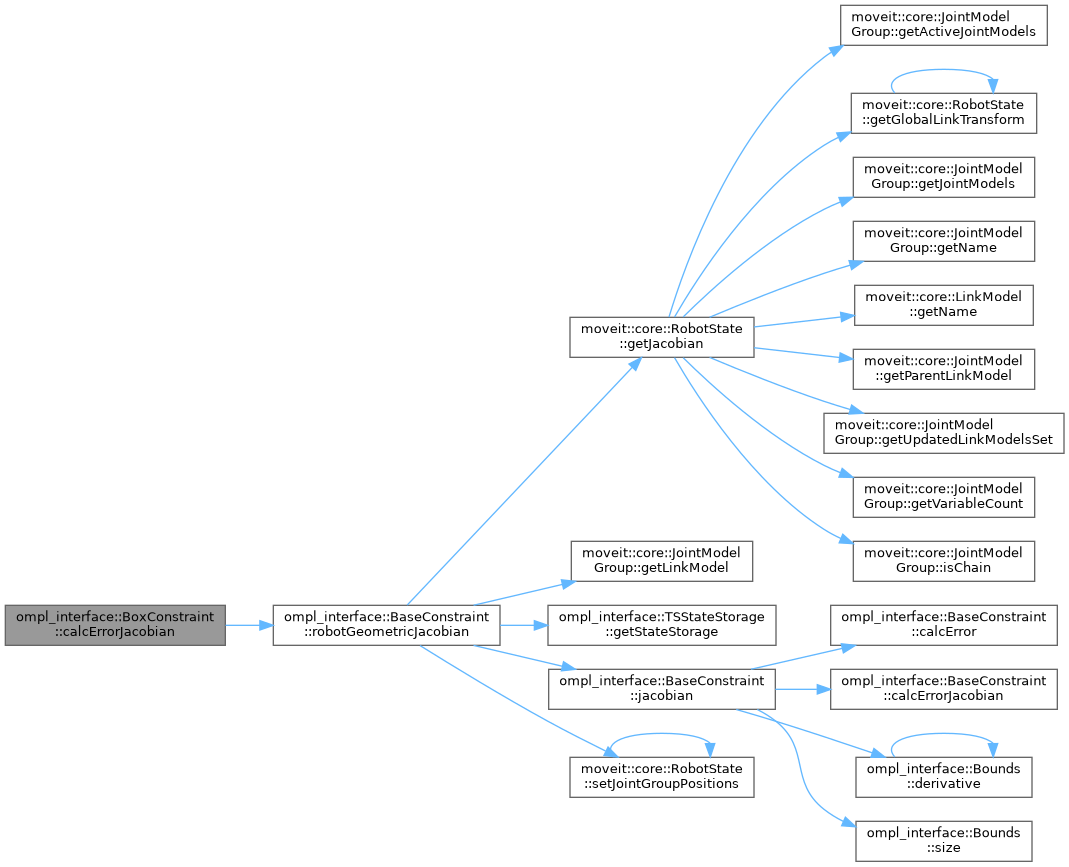## ◆ parseConstraintMsg()

 void ompl_interface::BoxConstraint::parseConstraintMsg ( const moveit_msgs::msg::Constraints & constraints )
overridevirtual

Parse bounds on position parameters from MoveIt's constraint message.

This can be non-trivial given the often complex structure of these messages.

Implements ompl_interface::BaseConstraint.

Definition at line 197 of file ompl_constraints.cpp.

Here is the call graph for this function: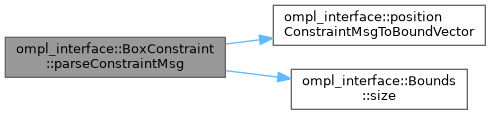The documentation for this class was generated from the following files: